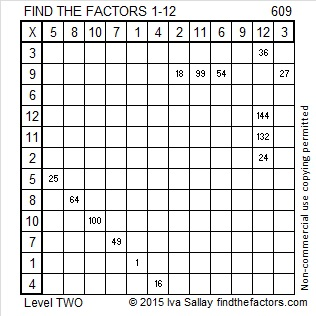# 609 and Level 2

609 is the hypotenuse of the Pythagorean triple 420-441-609. What is the greatest common factor of those three numbers?

21 x 29 = 609, both odd numbers from a factor pair for 609, and (29 – 21)/2 = 4. So since 4^2 = 16, we are 16 counting numbers away from a perfect square, in fact the next perfect square. Written mathematically that is (25-4)(25 +4) = (25^2) – (4^2).

609 is the 11th strobogrammatic number which means it looks like the same number upside-down because it uses only the digits 0, 1, 6, 8, and 9.

There are other numbers that don’t look the same upside-down but look the same reflected in a mirror. Check out teachfuthermaths for a fun puzzle about some of those numbers.

Print the puzzles or type the solution on this excel file: 12 Factors 2015-09-07

—————————————————————————————————

• 609 is a composite number.
• Prime factorization: 609 = 3 x 7 x 29
• The exponents in the prime factorization are 1, 1, and 1. Adding one to each and multiplying we get (1 + 1)(1 + 1)(1 + 1) = 2 x 2 x 2 = 8. Therefore 609 has exactly 8 factors.
• Factors of 609: 1, 3, 7, 21, 29, 87, 203, 609
• Factor pairs: 609 = 1 x 609, 3 x 203, 7 x 87, or 21 x 29
• 609 has no square factors that allow its square root to be simplified. √609 ≈ 24.677925.—————————————————————————————————